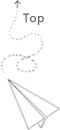# Resample and interpolation

manipulate the data

sampling

### Decompose data into blocks

hexiles

https://vtk.org/doc/nightly/html/classvtkProbeFilter.html#details

### VTK operation

probe

vtkProbFilter (maybe this can avoid the case that the original data is not the uniformgrid?) the source code is located at Filters/Core/vtkProbeFilter.h

Find the cell and then use the cell’s interpolation function, there are multiple types of the cell

We need to have a good understanding about the vtk cell type

This examples shows all the types of the vtkCell Type

https://kitware.github.io/vtk-examples/site/Cxx/GeometricObjects/LinearCellDemo/

Even the vtkLine (VTK/Common/DataModel/vtkLine.cxx) is an concrete example of the vtkCell (VTK/Common/DataModel), for each cell, there are EvaluateLocation and EvaluatePosition function and InterpolationFunctions, these are used to do some naive interpolation.

The dedicated point interpolation function use the different approch compared with the default one implemented by the cell operations here.

iso-parametric interpolation functions

Other types of interpolation do not have the operation of finding the cell, it just sample the points from cloud points, such as the example that use the PointInterpolator (the inputs is the list of points)

There are still some confusions about these operations, what are parametric coordinates? how to compute the weight etc

In the vtk text book here

There are some explanations about the VTK parametric coordinates

It is important to understand the meaning of the parametric coordinates in the VTK context. This coordinates are used to represents the position within the cell, its range is between 0 and 1.

Global coordinates

Local coordinates
To specify a location within the primary cell, we use geometric coordinates. These geometric coordinates, or parametric coordinates, are coordinates “natural” or canonical to the particular topology and dimension of a cell.

Every cell type will have its own parametric coordinate system

8-10 shows a 3d case, which is tr-linear iterpolation

using the vtkLine as an example, which is a concrete implementation of the vtkCell

DistanceToLine(get the parametric coordinates)
The input is the endpoint of the line and a point. The output is the distance from that point to the line and the cloestpoint to that dedicated point. The parametric coordinates is also returned here.

EvaluatePosition
This function calls the DistanceToLine to get necessary information.
It returns the if the associated point is in the line or out of the line. This function also compute the weight to interpolate the dedicated point based on givin points (the weight for p1 and p2 in this example)

EvaluateLocation

Then in actual probe operation, the pointData can use these weight values to construct the interpolated values.

This is an example code to show how the prob filter works, the coars grid is the original one and then we create a fine granularity grid based on it and use the prob to sample the data accordingly.

### PointCloud

For the case that there is no cell, how could we do that? Assuming the input the data is just a point cloud. In this case, we can use the vtkPointInterpolator.

The form for using the filter is similar to the previous one, the good thing is that we can switch different interpolation kernel in this example.

This example shows that how to set the inerp kernel, this is different since the input is supposed to be the points clouds, which is more flexible compared with the previous one for cellset.

## 推荐文章